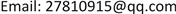﻿ 无人机影像误差分析和改善方法研究 Study on Image Error Analysis and Improvement Method of UAV

Computer Science and Application
Vol. 08  No. 09 ( 2018 ), Article ID: 26905 , 6 pages
10.12677/CSA.2018.89155

Study on Image Error Analysis and Improvement Method of UAV

Naiqiang Li, Chanjuan Liu

Survey Engineering Institute of Jiangsu Province, Nanjing JiangsuReceived: Sep. 1st, 2018; accepted: Sep. 14th, 2018; published: Sep. 21st, 2018ABSTRACT

In recent years, one of the developing trends of remote sensing is unmanned aerial vehicle (UAV) remote sensing. Unmanned aerial vehicle (UAV) remote sensing technology has become a powerful means to improve the potential of Surveying and mapping results, and is a shortcut to enhance the ability of Surveying and mapping emergency support. It is an important way to obtain remote sensing data, and it can obtain large scale and high resolution images, and has the advantages of strong image timeliness, strong pertinence, high flexibility in executing tasks and low running cost. The geometric position, size, shape and azimuth of the image in the image can be changed by the geometric position of the image pixel, such as the extrusion, extension, offset and distortion that occur in the image pixel of the unmanned aerial vehicle (UAV). It’s getting rid of it. In this paper, the causes of UAV image errors, the principles of geometric error correction, and the resampling of digital images are discussed in detail.

Keywords:Unmanned Aerial Vehicle, Image, Error, Analysis, Correction1. 引言

2. 无人机影像误差分析

2.1. 镜头畸变

2.2. 地形起伏引起的像点位移

2.3. 地球曲率引起的像点位移

2.4. 大气折射引起的像点位移

3. 几何误差校正

3.1. 数字影像的几何校正原理

$\left[\begin{array}{l}x\\ y\end{array}\right]=\rho \left[\begin{array}{cc}\mathrm{cos}\theta & \mathrm{sin}\theta \\ -\mathrm{sin}\theta & \mathrm{cos}\theta \end{array}\right]\left[\begin{array}{c}X+{X}_{0}\\ Y+{Y}_{0}\end{array}\right]$ (1.1)

$\begin{array}{l}x={a}_{11}X+{a}_{12}Y+{a}_{13}\\ y={a}_{21}X+{a}_{22}Y+{a}_{23}\end{array}\right\}$ (1.2)

$\left\{\begin{array}{l}x={a}_{10}+{a}_{11}X+{a}_{12}Y+{a}_{13}{X}^{2}+{a}_{14}XY+{a}_{15}{Y}^{2}+\cdots \cdots \\ y={a}_{20}+{a}_{21}X+{a}_{22}Y+{a}_{23}{X}^{2}+{a}_{24}XY+{a}_{25}{Y}^{2}+\cdots \cdots \end{array}$ (1.3)

3.2. 直接线性变换法

3.3. 多项式法

4. 数字影像的重采样

4.1. 最邻近像元法

$\begin{array}{l}I\left(P\right)=I\left(N\right)\\ {x}_{N}=INT\left(x+0.5\right)\\ {y}_{N}=INT\left(y+0.5\right)\end{array}$ (1.4)

4.2. 双线性内插法

$I=\left(1-\Delta x\right)\left(1-\Delta y\right)+\left(1-\Delta x\right)\Delta y{I}_{12}+\Delta x\left(1-\Delta y\right){I}_{12}+\Delta x\Delta y{I}_{22}$ (1.5)

4.3. 双三次卷积法

$h\left(x\right)=\left\{\begin{array}{l}{|x|}^{3}-2{|x|}^{2}+1,0\le |x|<1\\ -{|x|}^{3}+5{|x|}^{2}-8|x|+4,1\le |x|<2\\ 0,|x|\ge 2\end{array}$ (1.6)

5. 结语

Study on Image Error Analysis and Improvement Method of UAV[J]. 计算机科学与应用, 2018, 08(09): 1423-1428. https://doi.org/10.12677/CSA.2018.89155

1. 1. 王一, 胡莘, 赵莹芝. 全局一致性优化的无人机大倾角影像相对定向[J]. 测绘科学技术学报, 2017, 34(4): 382-386.

2. 2. 赵俊峰, 代亚贞, 范玉茹. 无人机影像快速拼接改进[J]. 测绘与空间地理信息, 2016, 39(9): 182-185.

3. 3. 郭忠磊, 赵志勇, 滕惠忠, 申家双, 张靓. 无人机影像自检校光束法区域网平差精度分析[J]. 海洋测绘, 2016, 36(2): 75-78.

4. 4. 杜娟, 薛武, 赵蓓蕾. 大规模无人机遥感影像快速区域网平差[J]. 现代防御技术, 2018, 46(4): 107-112, 168.

5. 5. 李炜宏. 浅析控制点布设对无人机影像空三精度的影响[J]. 中国新技术新产品, 2018(14): 5-6.

6. 6. 李大军, 孙涛, 郭丙轩, 牛科科, 梁跃刚. 一种基于倾斜影像的多片测图技术[J]. 测绘通报, 2018(7): 83-87.

7. 7. 张明娟. 无人机像控布设对影像精度的影响[J]. 青海国土经略, 2018(1): 67-69.